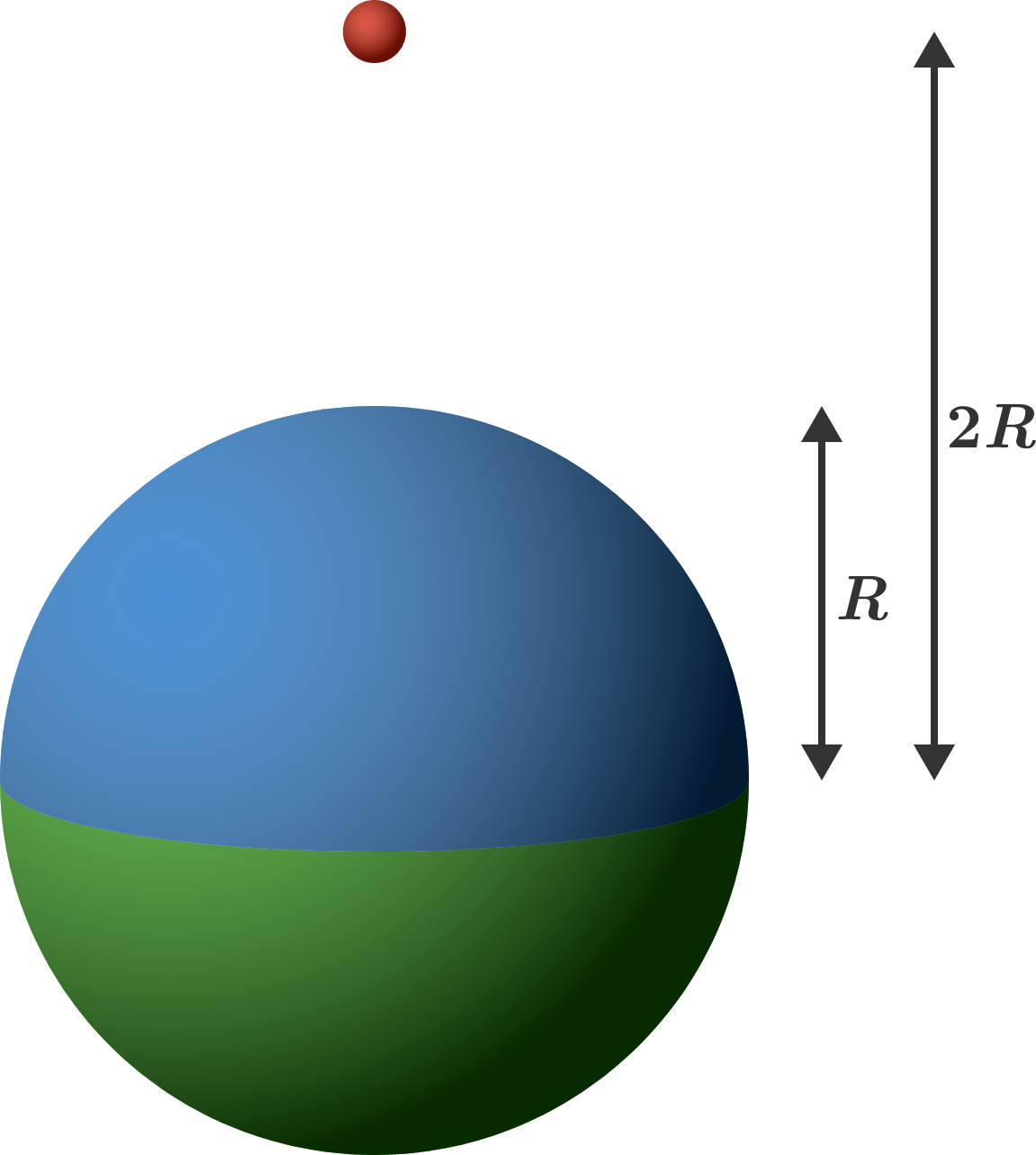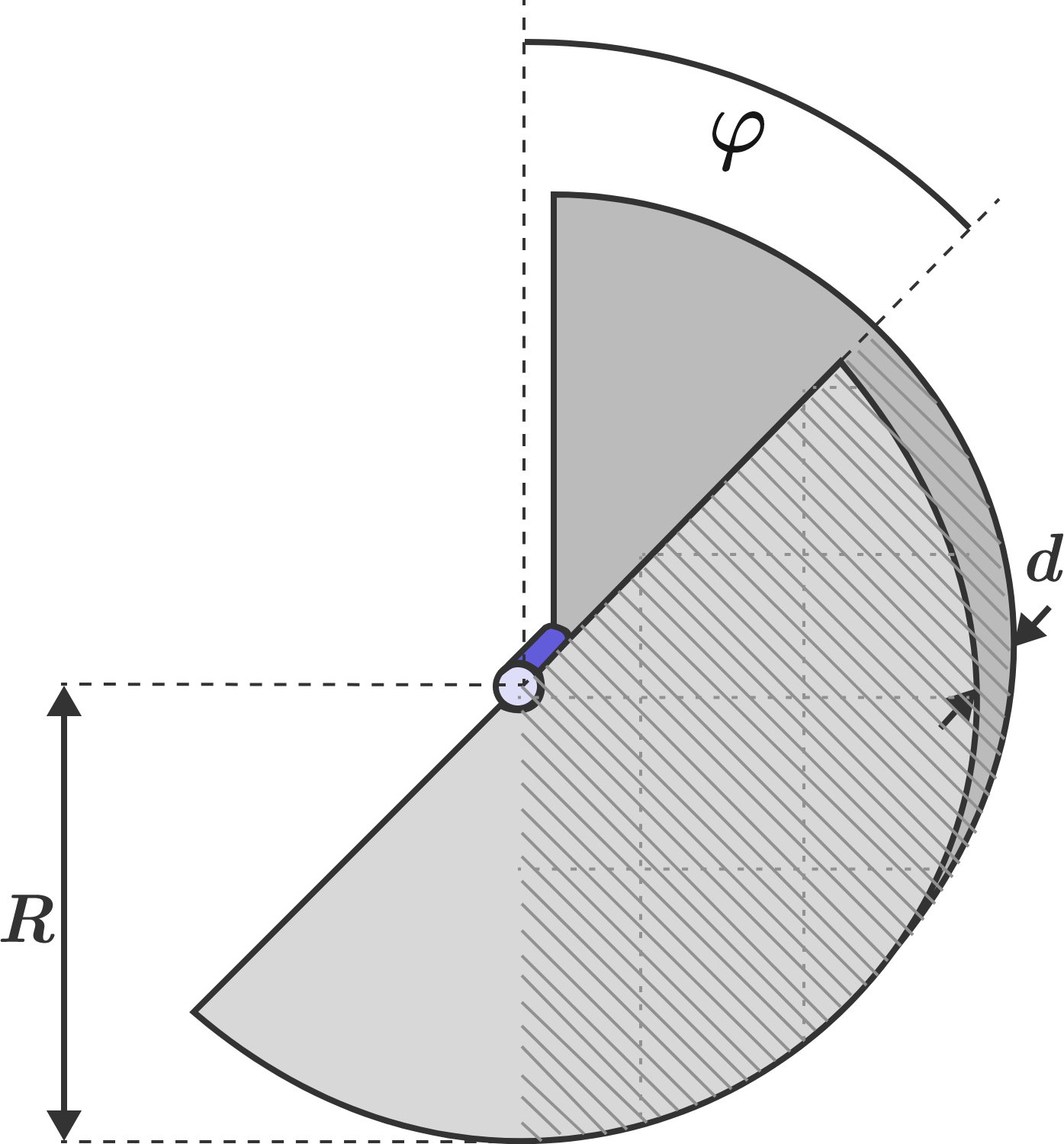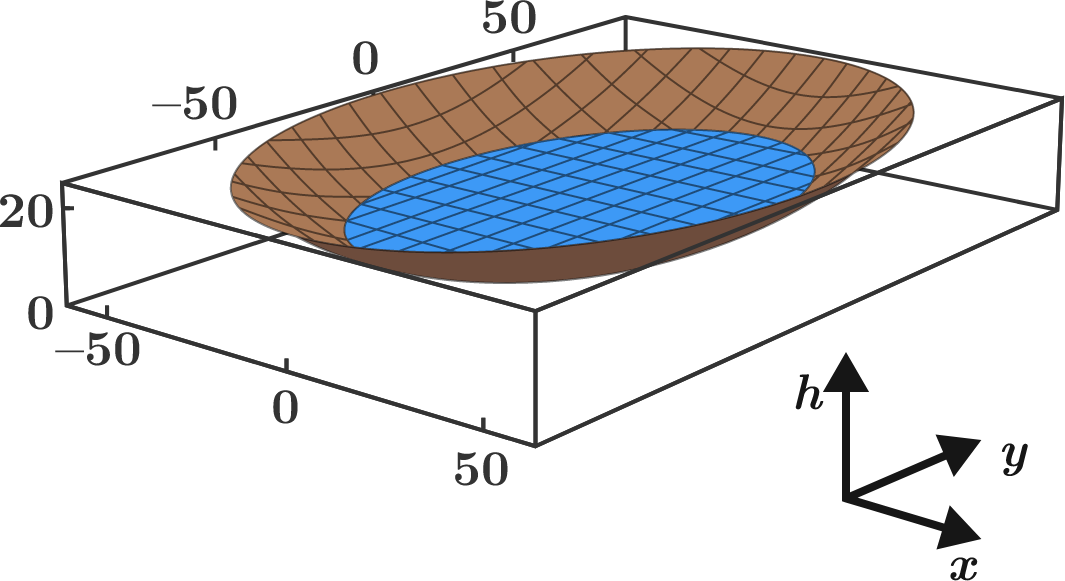# Problems of the Week

Contribute a problem

# 2017-11-27 AdvancedThe center of a solid sphere of radius $R$ is located a distance of $2R$ from a point-particle.

Approximately what percentage of the gravitational force felt by the point-particle is due to the blue half of the sphere?


Note: There is no ambient gravitational field.

Danielle, Lilliana, and Melody play a fighting video game in tournament mode. In this mode, two players play a match, and the winner of the match plays a new match against the player who was sitting out. This continues until a player wins two matches in a row. Danielle and Lilliana play the first match.

If they are all equally skilled at the game, then what is the probability that Melody will win the tournament?A variable capacitor consists of two metal semicircles of radius $R$ with vertical separation $d.$ The capacitor is charged when $\varphi=0,$ and is then disconnected from the voltage source. The discs are then rotated through the angle $\varphi = 90^\circ.$

How does the rotation change the energy that's stored in the capacitor?

The height profile of a valley basin can be described by the two-dimensional parabolic function$h(x, y) = \frac{x^2}{144\,\text{m}} + \frac{y^2}{324\,\text{m}}.$ Now the basin is filled by a rainstorm to a height of $h_0 = 12\,\text{m}.$ What is the volume of the resulting lake $($in $\text{m}^3)$ to the nearest integer?Hint: Find the shape of the cross-sectional areas enclosed by the equipotential lines $h(x, y) = z = \text{constant}$. The volume then results from the integral $\displaystyle V = \int_0^{h_0} A (z)\, dz$ over the cross-sectional area $A(z).$

$\mathscr{E} = \displaystyle \int_{0}^{\pi/2} \sqrt{1- \sin(2016 x) }\, dx$

Find $\mathscr{E}.$

Inspiration

Bonus: Generalize for $\displaystyle \int_{0}^{\pi/2} \sqrt{1- \sin(12n x) }\, dx$, where $n$ is a positive integer, and prove it.

×

Problem Loading...

Note Loading...

Set Loading...